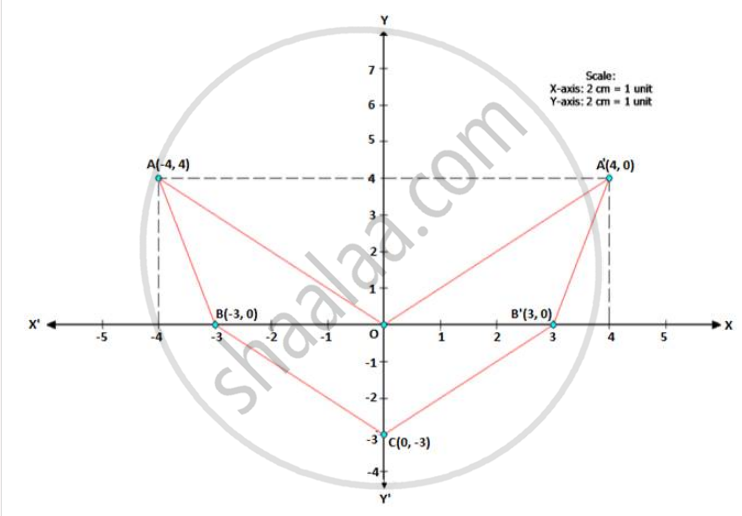# Plot the Points O(0,0), A(-4, 4), B(-3, 0) and C(0, -3). Reflect Points a and B on the Y-axis and Name Them A' and B' Respectively. Write Down Their Co-ordinates. Name the Figure Oabcb'A'. - Mathematics

Graph

Use graph paper for this question.

(Take 2 cm = 1 unit along both side x-axis and y-axis.)

Plot the points O(0,0), A(-4, 4), B(-3, 0) and C(0, -3).

1. Reflect points A and B on the y-axis and name them A' and B' respectively. Write down their co-ordinates.
2. Name the figure OABCB'A'.
3.  iii. State the line of symmetry of this figure. [Now symmetry is not in course]

#### Solution

1. A' = (4, 4) AND B' = (3, 0)
2. The figure is an arrow head.
3.  iii. The y-axis i.e. x = 0 is the line of symmetry of figure OABCB'A'.Concept: Invariant Points.
Is there an error in this question or solution?

#### APPEARS IN

Selina Concise Maths Class 10 ICSE
Chapter 12 Reflection
Exercise 12 (B) | Q 16 | Page 171